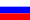# Vestnik КRAUNC. Fiz.-Mat. nauki. 2022. vol. 40. no. 3. P. 211-226. ISSN 2079-6641

Contents of this issue

Read Russian VersionMSC 65D30, 65D32

Research Article

Optimal quadrature formulas in the space \widetilde{W_2}^{(m,m-1)} of periodic functions

A. R. Hayotov¹², U. N. Khayriev¹

¹V. I. Romanovskiy Institute of Mathematics, Uzbekistan Academy of Sciences, 4b University str., Tashkent, 100174, Uzbekistan
²National University of Uzbekistan named after Mirzo Ulugbek, 4 University str., Tashkent, 100174, Uzbekistan
E-mail: hayotov@mail.ru,khayrievu@gmail.com

This paper is devoted to the process of finding the upper bound for the absolute error of the optimal quadrature formula in the space \widetilde{W_2}^{(m,m-1)} of real-valued, periodic functions. For this the extremal function of the quadrature formula is used. In addition, it is shown that the norm of the error functional for the optimal quadrature formula constructed in the space \widetilde{W_2}^{(m,m-1)} is less than the value of the norm of
the error functional for the optimal quadrature formula in the Sobolev space \widetilde{L_2}^{(m)}.

Key words: optimal quadrature formula, optimal coefficients, error of quadrature formula, the Hilbert space, the error functional, Fourier transform.

DOI: 10.26117/2079-6641-2022-40-3-211-226

Original article submitted: 30.07.2022

Revision submitted: 01.11.2022

For citation. Hayotov A. R., Khayriev U. N. Optimal quadrature formulas in the space \widetilde{W_2}^{(m,m-1)} of periodic functions. Vestnik KRAUNC. Fiz.-mat. nauki. 2022, 40: 3, 211-226.

DOI: 10.26117/2079-6641-2022-40-3-211-226

© Hayotov A. R., Khayriev U. N., 2022

Competing interests. The authors declare that there are no conflicts of interest regarding authorship and publication.

Contribution and Responsibility. All authors contributed to this article. Authors are solely responsible for providing the final version of the article in print. The final version of the manuscript was approved by all authors.

References

1. Baboş A., Acu A.M. Note on Corrected Optimal Quadrature Formulas in the Sense Nikolski, Applied Mathematics and Information Sciences an International Journal, 2015. vol. 9, no. 3, pp. 1231–1238.
2. Iserles A., Nørsett S.P. Efficient quadrature of highly oscillatory integrals using derivatives, Proc. R. Soc. A, 2005. vol. 461, pp. 1383–1399.
3. Boltaev A. K., Shadimetov Kh.M., Nuraliev F. A. The extremal function of interpolation formulas in W_2^{(2,0)} space,Vestnik KRAUNC. Fiz.-Mat. nauki, 2021. vol. 36, no. 3, pp. 123–132.
4. Burden A. M., Faires J. D., Burden R. L. Numerical analysis, 10th edition. Boston, Massachusetts: Cengage Learning, 2016.
5. Hayotov A. R., Milovanović G. V., Shadimetov Kh. M. Optimal quadratures in the sense of Sard in a Hilbert space, Applied Mathematics and Computation, 2015. vol. 259, pp. 637–653.
6. Hayotov A. R., Jeon S., Lee C-O., Shadimetov Kh. M. Optimal quadrature formulas for non-periodic functions in Sobolev space and its application to CT image reconstruction, Filomat, 2021. vol. 35, no. 12, pp. 4177–4195.
7. Hayotov A. R., Jeon S., Shadimetov Kh. M. Application of optimal quadrature formulas for reconstruction of CT images, Journal of Computational and Applied Mathematics, 2021. vol. 388, pp. 113313.
8. Hayotov A. R., Babaev S. S. Optimal quadrature formulas for computing of Fourier integrals in W_2^{(m,m-1)} space, AIP Conference Proceedings, 2021. vol. 2365, pp. 020021.
9. Hayotov A. R., Jeon S., Lee Ch.-O.On an optimal quadrature formula for approximation of Fourier integrals in the space L_2^{(1)} , Journal of Computational and Applied Mathematics, 2020. vol. 372, pp. 112713.
10. Hayotov A. R., Khayriev U. N. Construction of an optimal quadrature formula in the Hilbert space of periodic functions,Lobachevskii Journal of Mathematics, 2022.
11. Sard A. Best approximate integration formulas; best approximation formulas, Amer. J. Math., 1949. vol. 71, pp. 80–91.
12. Demidenko G. V., Vaskevich V. L. Selected Works of S. L. Sobolev. New York: Springer, 2006. 603 pp.
13. Milovanović G. V., StanićM.P. Numerical Integration of Highly Oscillating Functions /Analytic Number Theory, Approximation Theory and Special Functions. Berlin, Springer, 2014, pp. 613–649.
14. Milovanovi ć G. V. Numerical calculation of integrals involving oscillatory and singular kernels and some applications of quadratures, Comp. Math. Applic, 1998. vol. 36, no. 8, pp. 19–39.
15. Shadimetov Kh. M., Boltaev A. K., Parovik R. I. Construction of optimal interpolation formula exact for trigonometric functions by Sobolev’s method,Vestnik KRAUNC. Fiz.-Mat. nauki, 2022. vol. 38, no. 1, pp. 131–146.
16. Shadimetov Kh. M. Optimal lattice quadrature and cubature formulas in Sobolev spaces. Tashkent: Fan, 2019 (In Russian).
17. Shadimetov Kh. M. Weighted optimal quadrature formulas in a periodic Sobolev space, Uzbek Math. Journal, 1998. no. 2, pp. 76–86.
18. Shadimetov Kh. M..Weighted optimal cubature formulas in the periodic Sobolev space, Siberian Journal of Computational Mathematics, 1999. no. 2 , pp. 185–196.
19. Filon L. N. G.On a quadrature formula for trigonometric integrals, Proc. Roy. Soc., 1928. vol. 49, pp. 38–47.
20. Temme N. M. Special functions: An introduction to the classical functions of mathematical physics. New York: A Wiley-Interscience, 1996. 374 pp.
21. Bakhvalov N. S., Vasil’eva L. G. Evaluation of the integrals of oscillating functions by interpolation at nodes of Gaussian quadratures,USSR Computational Mathematics and Mathematical Physics, 1968. vol. 8, pp. 241–249 (In Russian).
22. Sobolev S. L., Vaskevich V. L. The Theory of Cubature Formulas. Dordrecht: Kluwer Academic Publishers Group, 1997.
23. Nikolskii S. M. Quadrature formulas. Moscow: Nauka, 1988 (In Russian).
24. Olver S. Numerical Approximation of Highly Oscillatory Integrals, PhD dissertation: University of Cambridge, 2008.
25. Babaev S. S., Hayotov A. R., Khayriev U. N.On an optimal quadrature formula for approximation of Fourier integrals in the space W_2^{(1,0)}, Uzbek Mathematical Journal, 2020. no. 2, pp. 23–36.
26. Xu Z. Milovanović G. V., Xiang S. Efficient computation of highly oscillatory integrals with Henkel kernel, Appl. Math. Comp., 2015. vol. 261, pp. 312–322.

Hayotov Abdullo Rahmonovich – D. Sci. (Phys. & Math.), Professor, Head of the Laboratory of Computational Mathematics, V.I.Romanovskiy Institute of Mathematics, Uzbekistan Academy of Sciences, Tashkent, Uzbekistan, ORCID 0000-0002-2756-9542.

Khayriev Umedjon Narmon ugli – PhD Student at the laboratory of Computational Mathematics, V. I. Romanovskiy Institute of Mathematics, Uzbekistan Academy of Sciences, Tashkent, Uzbekistan Laboratory of Computational Mathematics, ORCID 0000-0003-2156-3745.